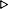ToC DocOverview CGDoc RelNotes Index PermutedIndex
Allegro CLANSI Common Lisp5 Data and Control Flow5.3 Dictionary of Data and Control Flow

 5.3.43 if Special Operator

Syntax:
if test-form then-form [else-form]{result}*

Arguments and Values:
Test-form - a form.

Then-form - a form.

Else-form - a form. The default is nil.

results - if the test-form yielded true, the values returned by the then-form; otherwise, the values returned by the else-form.

Description:
if allows the execution of a form to be dependent on a single test-form.

First test-form is evaluated. If the result is true, then then-form is selected; otherwise else-form is selected. Whichever form is selected is then evaluated.

Examples:
``` (if t 1)1
(if nil 1 2)2
(defun test ()
(dolist (truth-value '(t nil 1 (a b c)))
(if truth-value (print 'true) (print 'false))
(prin1 truth-value)))TEST
(test)TRUE TFALSE NILTRUE 1TRUE (A B C)NIL
```

``` (if test-form then-form else-form)